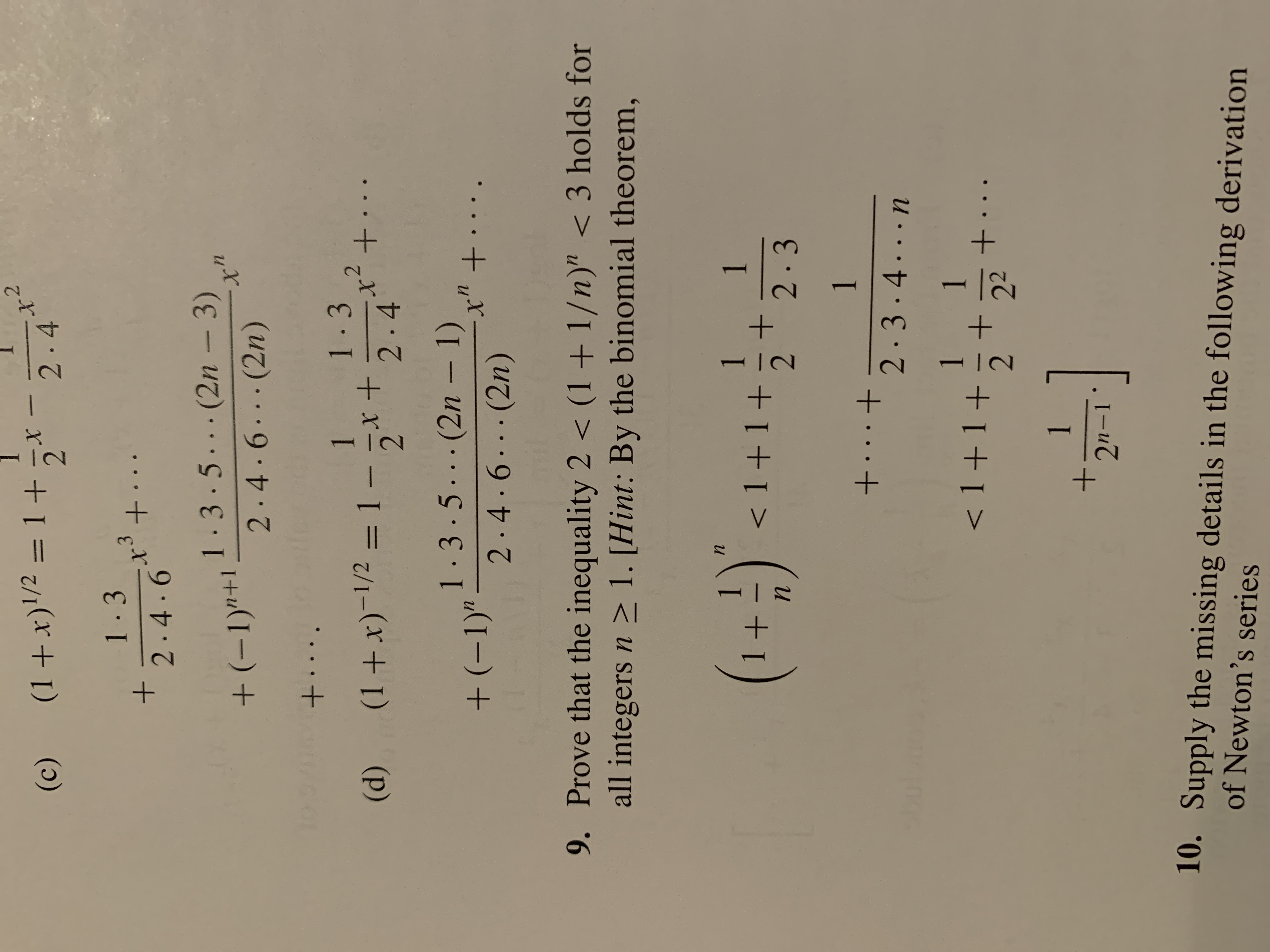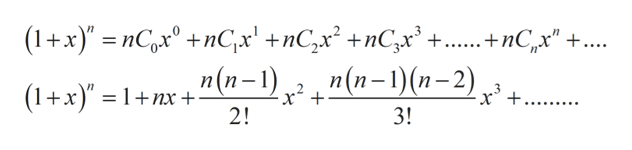# 2.4(1+x)'/2 = 1 +5*(c)1-33.+.2.4.61-3.5...(2n – 3)+(-1)"+12.4.6...(2n)To +....1-31x²+(1+x)-1/2 = 1 - x +2.4· ·(d)%3D1.3.5...(2n – 1)+ (-1)"-.t.x" +....2.4.6...(2n)9. Prove that the inequality 2 < (1+ 1/n)" < 3 holds forall integers n > 1. [Hint: By the binomial theorem,(1+)".1< 1+1+12.31+...+2.3.4...n1< 1 +1+1+..221.2n-110. Supply the missing details in the following derivationof Newton's series

Question
2 views

8d:  use the binomial theorem to obtain the following series expansionshelp_outlineImage Transcriptionclose2.4 (1+x)'/2 = 1 +5* (c) 1-3 3. +. 2.4.6 1-3.5...(2n – 3) +(-1)"+1 2.4.6...(2n) To +.... 1-3 1 x²+ (1+x)-1/2 = 1 - x + 2.4 · · (d) %3D 1.3.5...(2n – 1) + (-1)" - .t. x" +.... 2.4.6...(2n) 9. Prove that the inequality 2 < (1+ 1/n)" < 3 holds for all integers n > 1. [Hint: By the binomial theorem, (1+)". 1 < 1+1+ 1 2.3 1 +...+ 2.3.4...n 1 < 1 +1+ 1 +.. 22 1. 2n-1 10. Supply the missing details in the following derivation of Newton's series fullscreen
check_circle

Step 1

Using the binomial theorem for the series expansion, here is ...help_outlineImage Transcriptionclose(1+х)" — пС)х" +nC;x' + пC,x* + пCx* +..... + nC, x" +... п(п-1), n(п-1)(п-2) (1+x)" = 1+nx + - x² + 2! +. ........ 3! fullscreen

### Want to see the full answer?

See Solution

#### Want to see this answer and more?

Solutions are written by subject experts who are available 24/7. Questions are typically answered within 1 hour.*

See Solution
*Response times may vary by subject and question.
Tagged in

### Math